TitleCollege Algebra
Answer/Discussion to Practice Problems
Tutorial 54A: SequencesAnswer/Discussion to 1a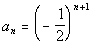;    1 < n < 5

 Basically, to find the nth term of a sequence works in the same fashion as function notation.  If you want to find the 3rd term, you are looking for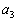, which means you plug in 3 for n into the given function. So, what are we going to plug in for n to find the 1st term?  If you said 1, give yourself a pat on the back.  What about the 2nd term?  I hope you said you would plug in 2 for n.   Since we have to go from 1 < n < 5, this means we need to find 5 terms and we will be plugging in 1, 2, 3, 4, and 5 for n. Let's see what we get:*1st term, n = 1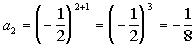*2nd term, n = 2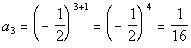*3rd term, n = 3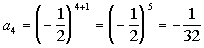*4th term, n = 4*5th term, n = 5

 Note how we had -1 raised to n, which changes value, and the signs of the terms alternated. The five terms of this sequence are 1/4, -1/8, 1/16, -1/32, and 1/64.Answer/Discussion to 2a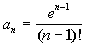This function contains a factorial. Let's see what we get for our first five terms: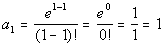*1st term, n = 1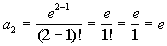*2nd term, n = 2*3rd term, n = 3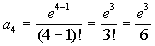*4th term, n = 4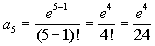*5th term, n = 5

 Now let's check out the twelfth term: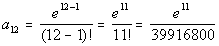*12th term, n = 12Answer/Discussion to 3a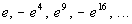Let's take a look at what is happening here:

Something that is always constant is that each term contains e.

There are also two things that change.

First let's look at the alternating signs:

 For it to have alternating signs, we need to have (-1) raised to a power that changes.  This means n, the term number is involved. The first term is positive, the second term is negative, the third positive, the fourth negative and so forth. When n is odd (1, 3, 5, ...), then the term is positive. When n is even (2, 4, 6, ...), then the term is negative.  So do you think we are going to have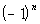or. If you saidyou are correct!!!  If n is odd, then this term will be positive.  If n is even, then this term will be negative.

We also have the exponent on each term.

Again we need to figure out the relationship between n and the exponent:

 When n is 1, the exponent is 1. When n is 2, the exponent is 4. When n is 3, the exponent is 9. When n is 4, the exponent is 16.

What do you think the relationship is?
It looks like the exponent is always n squared.

Putting it together, the formula for the nth term is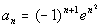.

Sometimes you have to play around with it before you get it just right.  You can always check it by putting in the n values and seeing if you get the given sequence.

This one does check.Answer/Discussion to 4a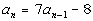;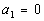We are giving the first term,.  Using that we can find the second term and so forth. Let's see what we get for our first three terms:*2nd term, n = 2*3rd term, n = 3

 Since this is a recursive formula, in order to the fifth term, we need to find the fourth term: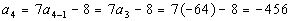*4th term, n = 4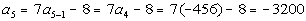*5th term, n = 5

Last revised on May 16, 2011 by Kim Seward.
All contents copyright (C) 2002 - 2011, WTAMU and Kim Seward. All rights reserved.